# Hashing

Updated:

⚠️ This post was created when I was in high school and is no longer maintained.

( hereby, we temporarily ignore the satellite data and only care about the key. )

Although searching for an element in a hash table can take as long as searching for an element in a linked list— O(n) time in the worst case—in practice, hashing performs extremely well. Under reasonable assumptions, the average time to search for an element in a hash table is O(1). —— CRLS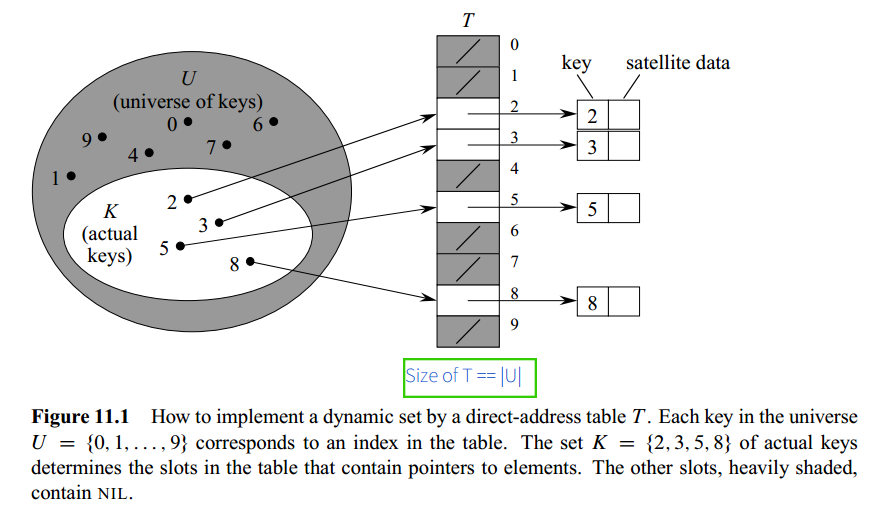### 1.1 Intuition

We use keys themselves as the hash table index.

Direct addressing is a simple technique that works well when the universe U of keys is reasonably small. Suppose that an application needs a dynamic set in which each element has a key drawn from the universe U = {0, 1, 2, ..., m-1}, where m is not too large. We shall assume that no two elements have the same key.

### 1.2 Pseudocode

algorithm DirectAddressSearch(T, k):
return T[k]

T[x.key] := x

T[x.key] := NIL


### 1.3 Downsides

• If the universe U is large, storing a table T of size |U| may be impractical;

• The set K of keys actually stored may be so small relative to U that most of the space allocated for T would be wasted.

Tips:

• Rather than storing an element’s key and satellite data in an object external to the direct-address table, with a pointer from a slot in the table to the object, we can store the object in the slot itself, thus saving space.
• It is often unnecessary to store the key of the object since if we have the index of an object in the table, we have its key.
• If keys are not stored, however, we must have some way to tell whether the slot is empty.

## 2. Hash tables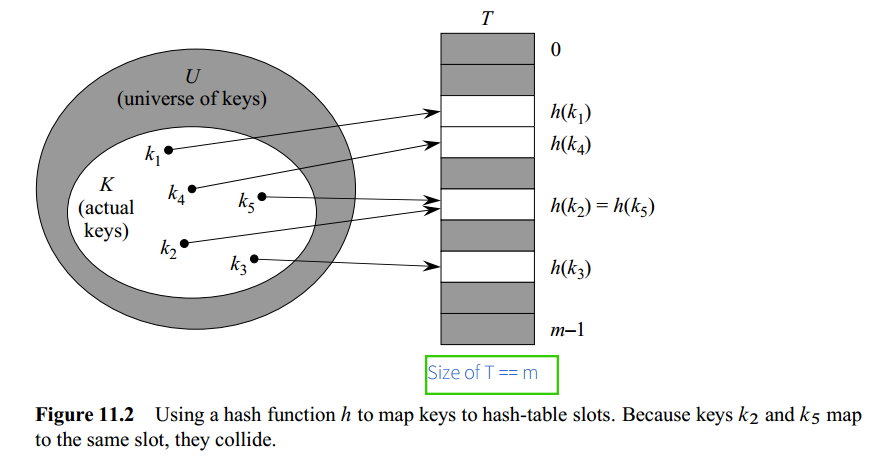1. Intuition: With direct addressing, an element with key k is stored in slot k. With hashing, this element is stored in slot h(k).
2. We use a hash function h to compute the slot from the key k.
• Here, h maps the universe U of keys into the slots of a hash table T[0, 1, ..., m-1]: $h: U \rightarrow~ \{0, 1, ..., m-1\}$
3.  the size m of the hash table is typically much less than U .
4. We say that an element with key k hashes to slot h(k); we also say that h(k) is the hash value of key k.
5. Collision: two different keys hash to the same slot.
• Make hash function h appear to be “random”;

The very term “to hash,” evoking images of random mixing and chopping, captures the spirit of this approach.

• This a Pigeon Hole Problem: |U| >> m

there must be at least two keys that have the same hash value; avoiding collisions altogether is therefore impossible.

## 3. Collision resolution by chaining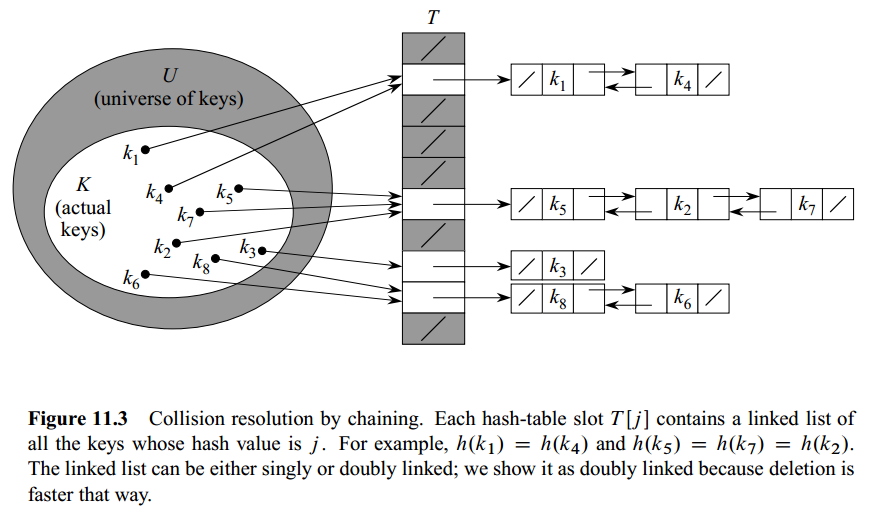1. Pseudocode
algorithm ChainedSearch(T, k):
search for an element with key k in list T[h(k)]

algorithm ChainedInsert(T, x):
insert x at the head of list T[h(k)]

algorithm ChainedDelete(T, x):
delete x from list T[h(k)]

1. Time complexity

2.1 ChainedInsert() and ChainedDelete(): T(n) = O(n)

tips: For the sake of efficiency, the chain should be doubly linked-list. If they are singly linked-list, we have to search the predecessor of the target node before delete because the previous node’s next pointer is not available via the target node.

2.2 ChainedSearch(): (n elements, m slots)

• Load factor α for T is n/m – the average number of elements stored in a chain.
• Worst-case: all n keys hash to the same slot, creating a list of length n: Θ(n+1)
• Average-case:

depends on how well the hash function h distributes the set of keys to be stored among the m slots

Simple uniform hashing Assumption: any given element is equally likely to hash into any of the mslots, independently of where any other element has hashed to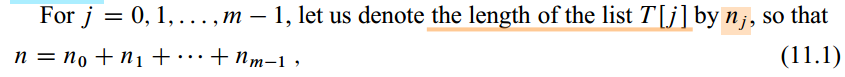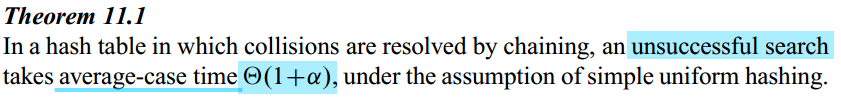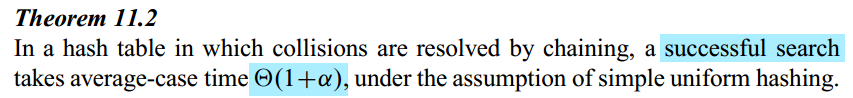## 4. Hash Functions

A good hash function satisfies (approximately) the assumption of simple uniform hashing: each key is equally likely to hash to any of the m slots, independently of where any other key has hashed to.

We typically have no way to check this condition, since we rarely know the probability distribution from which the keys are drawn. Moreover, the keys might not be drawn independently. —— CRLS

### 4.1 The division method

m should not be a power of 2, since if m = 2^p^, then h(k) is just the p lowest-order bits of k.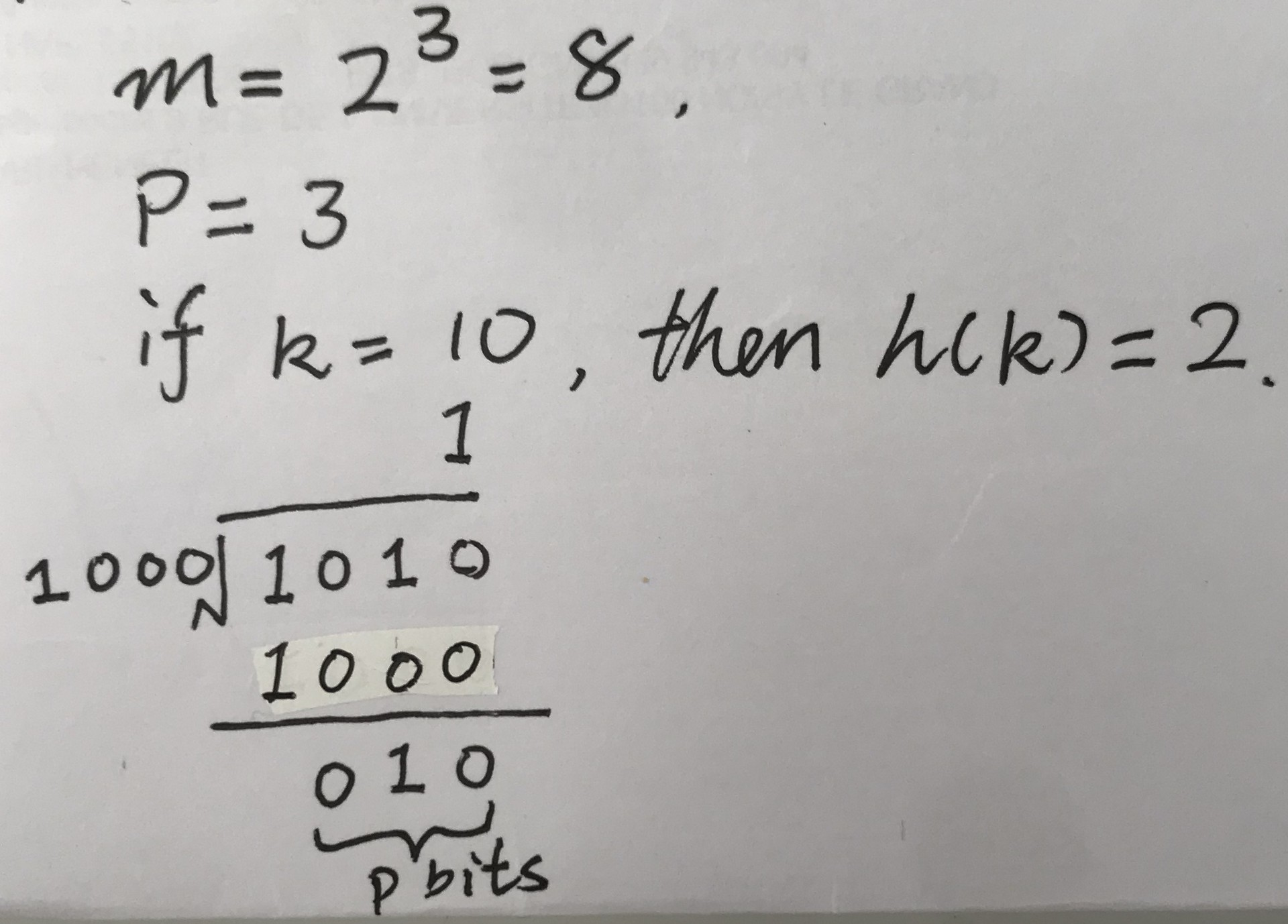### 4.2 The multiplication method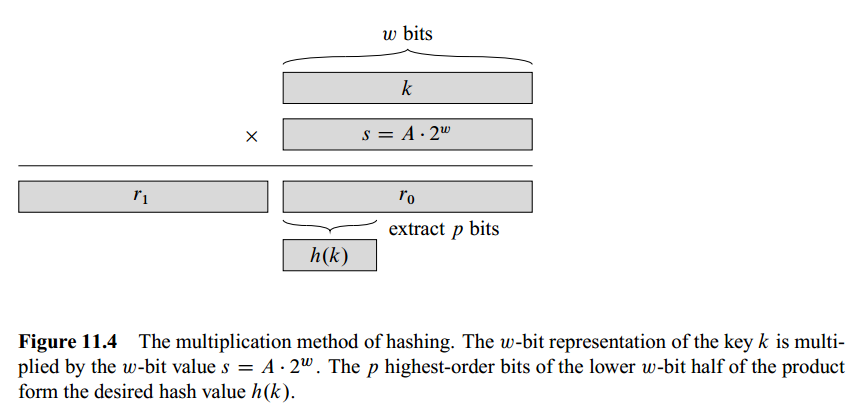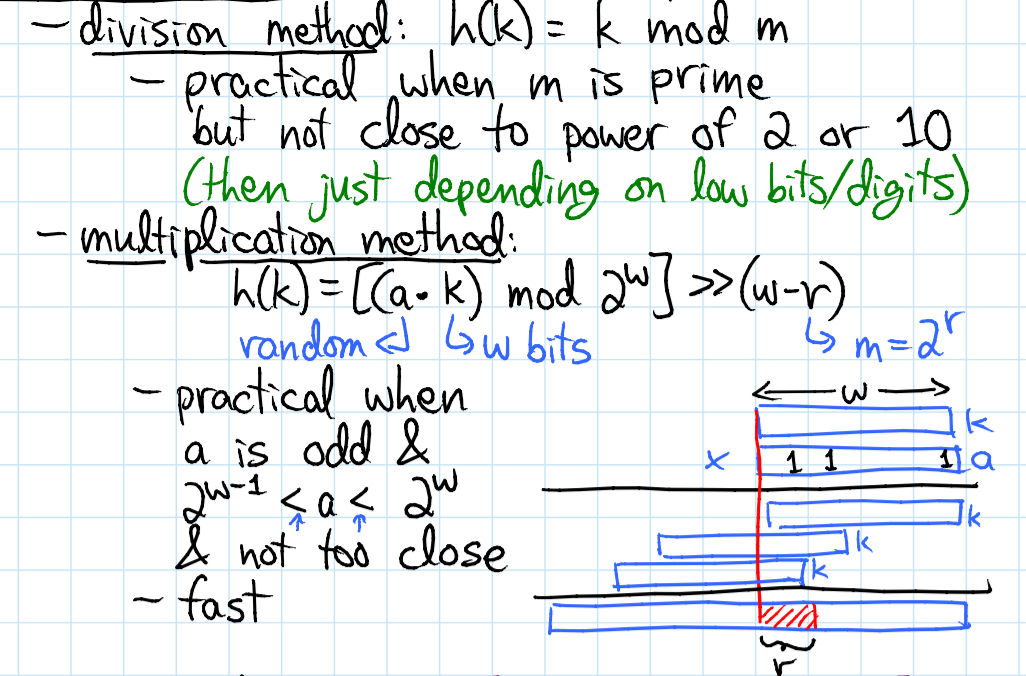## 5. Collision resolution by Open Addressing (do not limit the number of addresses)

In open addressing, all elements occupy the hash table itself. That is, each table entry contains either an element of the dynamic set or NIL.

No lists and no elements are stored outside the table, unlike in chaining.

The load factor α can never exceed 1 (There is always a more or equal number of slots than elements)

### 5.1 Pseudocode

algorithm HashInsert(T, k):
i := 0
While i != m do
j = h(k, i)
if T[j] == NIL or T[j] == Deleted then
T[j] = k
return j
i += 1
Throw error "hash table overflow (full)"

algorithm HashSearch(T, k):
i := 0
do
j = h(k, i)
if T[j] == k:
return j
i += 1
While T[j] != NIL or i != m --> Ingore Deleted so that we won't return incorrectly

return NIL

algorithm HashDelete(T, k):
T[HashSearch(T, k)] = Deleted



### 5.2 Probe (successively examine):

To determine which slots to probe, we extend the hash function to include the probe number (starting from 0) as a second input.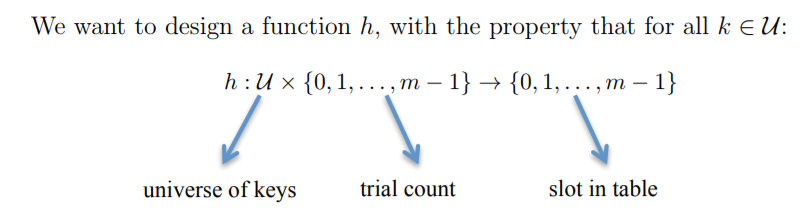With open addressing, we require that for every key k, the probe sequence:

$\rang h(k, 1), h(k, 2), ... , h(k, m-1) \rang$

is a permutation of 0, 1, … , m − 1. i.e. if I keep trying h(k, i) for increasing i, I will eventually hit all slots of the table.

Tip: the indices of the hash table start from 0 and this is why the range of probing is [0 ~ m-1]

### 5.2.1 Linear probing

We regard the ordinary hashing function:

$h: U \rightarrow~ \{0, 1, ..., m-1\}$

as an auxiliray hash function h’

• Linear probing hash function: (like street parking) $h(k, i) = (h'(k) +i) ~ mod ~ m$

• Problem: Primary Clustering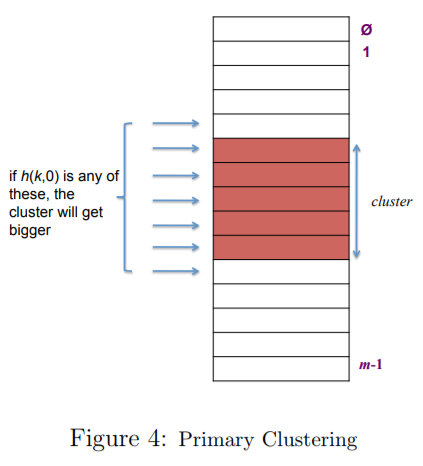### 5.2.2. Double Hashing:

( to tackle the Primary Clustering problem )

$h(k, i) =(h1(k) +i·h2(k)) ~ mod ~ m$ (where h1(k) and h2(k) are two ordinary hash functions.)

Tips: it actually hits all slots (permutation) if h2(k) is relatively prime to m for all k

  h1(k) + i · h2(k) mod m
= h1(k) + j · h2(k) mod m
⇒ m divides (i − j)


### 5.2.3 Analysis

Suppose we have used open addressing to insert n items into table of size m. Under the uniform hashing assumption the next operation has expected cost of $T(n)\le\cfrac{1}{1-\alpha} ~~where~~ (\alpha = n/m \lt1) \\ = O(1/(1-\alpha)) ~~~~~~~~~~~~~~~~~~~~ \\\\for~Insert(),~Search(),~Delete()$ (proof: see CLRS)

Example:

α = 90% ⇒ 10 expected probes α = 50% ⇒ 2 expected probes